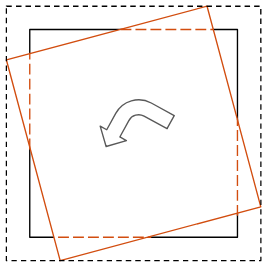A high-performance general-purpose compute library

Rotate an input image or array. More...

## Functions

AFAPI array rotate (const array &in, const float theta, const bool crop=true, const interpType method=AF_INTERP_NEAREST)
C++ Interface for rotating an image.

AFAPI af_err af_rotate (af_array *out, const af_array in, const float theta, const bool crop, const af_interp_type method)
C Interface for rotating an image.

## Detailed Description

Rotate an input image or array.

The rotation is done counter-clockwise, with an angle theta (in radians), using a specified method of interpolation to determine the values of the output array. Six types of interpolation are currently supported:

Since the output image still needs to be an upright box, crop determines how to bound the output image, given the now-rotated image. The figure below illustrates the effect of changing this parameter.Effect of crop parameter on the output

Here, the original image is represented by the innermost box with the solid black and dashed orange lines, and the (theoretical) rotated image is the box with the solid orange lines. If crop is true, then the output image's dimensions will stay the same as the original image's, but the rotated image's portions outside the dashed orange lines will be cropped, and the rest of the output image (the area between the solid black and solid orange lines) will be filled with zeros. However, if crop is false, then the output image's dimensions might get bigger (as shown in this illustration), as represented by the outermost box with dashed black lines. This change in dimensions is necessary to accommodate all of the rotated image's data. The remainder of the output image will be filled with zeros, as represented by the area between the solid orange lines and dashed black lines. Note that the new dimensions in general (beyond this illustration) will be greater than or equal the original image's dimensions when crop is false.

## ◆ af_rotate()

 AFAPI af_err af_rotate ( af_array * out, const af_array in, const float theta, const bool crop, const af_interp_type method )

C Interface for rotating an image.

Parameters
 [out] out will contain the image in rotated by theta [in] in is input image [in] theta is the degree (in radians) by which the input is rotated [in] crop if true the output is cropped original dimensions. If false the output dimensions scale based on theta [in] method is the interpolation type (Nearest by default)
Returns
AF_SUCCESS if the color transformation is successful, otherwise an appropriate error code is returned.

## ◆ rotate()

 AFAPI array rotate ( const array & in, const float theta, const bool crop = true, const interpType method = AF_INTERP_NEAREST )

C++ Interface for rotating an image.

Parameters
 [in] in is input image [in] theta is the degree (in radians) by which the input is rotated [in] crop if true the output is cropped original dimensions. If false the output dimensions scale based on theta [in] method is the interpolation type (Nearest by default)
Returns
the image rotated by theta